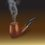# On divergence of Series

In this note I will prove that the series $${ \left( { S }_{ n } \right) }_{ n\ge 1 }$$ given by ${ S }_{ n }=\sum _{ r=1 }^{ n }{ \frac { 1 }{ r } } \forall n\in N$ is divergent. If we can find out a divergent sub-series of $${ \left( { S }_{ n } \right) }_{ n\ge 1 }$$, we can say that $${ \left( { S }_{ n } \right) }_{ n\ge 1 }$$ diverges. For that we consider the sub-series $${ \left( { S }_{ { 2 }^{ n } } \right) }_{ n\ge 1 }$$. We then observe that ${ S }_{ { 2 }^{ n } }=\sum _{ r=1 }^{ { 2 }^{ n } }{ { \frac { 1 }{ r } } } \\ =1+\frac { 1 }{ 2 } +\left( \frac { 1 }{ 3 } +\frac { 1 }{ 4 } \right) +\left( \frac { 1 }{ 5 } +\frac { 1 }{ 6 } +\frac { 1 }{ 7 } +\frac { 1 }{ 8 } \right) +...+\left( \frac { 1 }{ { 2 }^{ n-1 }+1 } +\frac { 1 }{ { 2 }^{ n-1 }+2 } +...+\frac { 1 }{ { 2 }^{ n } } \right) \\ >1+\frac { 1 }{ 2 } +\left( \frac { 1 }{ 4 } +\frac { 1 }{ 4 } \right) +\left( \frac { 1 }{ 8 } +\frac { 1 }{ 8 } +\frac { 1 }{ 8 } +\frac { 1 }{ 8 } \right) +...+\left( \frac { 1 }{ { 2 }^{ n } } +\frac { 1 }{ { 2 }^{ n } } +...\left( { 2 }^{ n-1 }\quad times \right) ...+\frac { 1 }{ { 2 }^{ n } } \right) \\ =1+\left( \frac { 1 }{ 2 } +\frac { 1 }{ 2 } +...\left( n\quad times \right) ...+\frac { 1 }{ 2 } \right) \\ =1+\frac { n }{ 2 }$ Hence, as the sequence $${ \left( 1+\frac { n }{ 2 } \right) }_{ n\ge 1 }$$ diverges, the sub-series $${ \left( { S }_{ { 2 }^{ n } } \right) }_{ n\ge 1 }$$ and hence the series $${ \left( { S }_{ n } \right) }_{ n\ge 1 }$$ diverges.Note by Kuldeep Guha Mazumder
5 years, 9 months ago

This discussion board is a place to discuss our Daily Challenges and the math and science related to those challenges. Explanations are more than just a solution — they should explain the steps and thinking strategies that you used to obtain the solution. Comments should further the discussion of math and science.

When posting on Brilliant:

• Use the emojis to react to an explanation, whether you're congratulating a job well done , or just really confused .
• Ask specific questions about the challenge or the steps in somebody's explanation. Well-posed questions can add a lot to the discussion, but posting "I don't understand!" doesn't help anyone.
• Try to contribute something new to the discussion, whether it is an extension, generalization or other idea related to the challenge.

MarkdownAppears as
*italics* or _italics_ italics
**bold** or __bold__ bold
- bulleted- list
• bulleted
• list
1. numbered2. list
1. numbered
2. list
Note: you must add a full line of space before and after lists for them to show up correctly
paragraph 1paragraph 2

paragraph 1

paragraph 2

[example link](https://brilliant.org)example link
> This is a quote
This is a quote
    # I indented these lines
# 4 spaces, and now they show
# up as a code block.

print "hello world"
# I indented these lines
# 4 spaces, and now they show
# up as a code block.

print "hello world"
MathAppears as
Remember to wrap math in $$ ... $$ or $ ... $ to ensure proper formatting.
2 \times 3 $2 \times 3$
2^{34} $2^{34}$
a_{i-1} $a_{i-1}$
\frac{2}{3} $\frac{2}{3}$
\sqrt{2} $\sqrt{2}$
\sum_{i=1}^3 $\sum_{i=1}^3$
\sin \theta $\sin \theta$
\boxed{123} $\boxed{123}$

Sort by:

could u pls explain me the second step

- 3 years, 8 months ago

They were doing something called the direct comparison test. Basically, they made a series where every term was the same or smaller than the terms of the original series, and showed that it diverged. Therefore, because a series smaller than the one given diverges, the bigger one must diverge.

- 3 years, 7 months ago